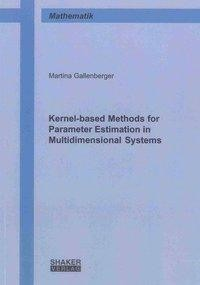Klimaneutrales UnternehmenFaire PreiseSchneller & kostenloser Versand
Gebrauchte & Neue Bücher
Keine Versandkosten

# Kernel-based Methods for Parameter Estimation in Multidimensional SystemsGebrauchte Bücher kaufen

Information
Das Buch befindet sich in einem sehr guten, unbenutzten Zustand.
Information
Das Buch befindet sich in einem sehr guten, gelesenen Zustand. Die Seiten und der Einband sind intakt. Buchrücken/Ecken/Kanten können leichte Gebrauchsspuren aufweisen.
Information
Das Buch befindet sich in einem guten, gelesenen Zustand. Die Seiten und der Einband sind intakt. Buchrücken/Ecken/Kanten können Knicke/Gebrauchsspuren aufweisen.
Information
Das Buch befindet sich in einem lesbaren Zustand. Die Seiten und der Einband sind intakt, jedoch weisen Buchrücken/Ecken/Kanten starke Knicke/Gebrauchsspuren auf. Zusatzmaterialien können fehlen.

Neues Buch oder eBook (pdf) kaufen

Information
Neuware - verlagsfrische aktuelle Buchausgabe.
Dieser Artikel steht derzeit nicht zur Verfügung!
Zustand : Neu
45,80 €

inkl. MwSt. zzgl. Versandkosten

Artikel zZt. nicht lieferbar

9783844021738

#### Die Studibuch PhilosophieWissen zu fairen Preisen, nachhaltig weitergeben.
Produktdetails mehr
 Einband: Kartoniert Seitenzahl: 110 Erschienen: 2013-09-01 Sprache: Englisch EAN: 9783844021738 ISBN: 3844021736 Reihe: Berichte aus der Mathematik Verlag: Shaker Verlag Gewicht: 162 g Auflage:
In this thesis, we show how kernel regularization approaches can be used for parameter estimation... mehr
Produktinformationen "Kernel-based Methods for Parameter Estimation in Multidimensional Systems"
In this thesis, we show how kernel regularization approaches can be used for parameter estimation in different multidimensional systems. We study this problem in the context of reproducing kernel Hilbert spaces of vector-valued functions. This also allows interpretation in terms of Gaussian processes and RBF approximation networks. The results of the thesis are the extension of estimation methods to the case of vector-valued data which includes the development of new approximation approaches or optimization criteria. Furthermore, we present a new model for the glucose-insulin regulatory system illustrating recent biological results. This model is used as a concrete example for the use of a RBF approximation network to estimate the solution of a system of differential equations. In the first chapter we summarize two basic concepts for estimating vector-valued functions based on a finite set of measurements. As this problem is ill-posed, we consider regularization approaches for function estimation. First, we present the main results of the theory of operator-valued reproducing kernels and corresponding reproducing kernel Hilbert spaces (RKHS) of vector-valued functions. We recall the one-to-one relationship of operator-valued kernels and RKHS, the concept of minimal norm interpolation in RKHS, and its extension to regularization approaches in a vector-valued setting. We then describe Gaussian process regression for learning vector-valued functions. We present the weight-space view using Bayesian inference and the corresponding function-space view based on the assumption that the predictive function is drawn from a vector-valued Gaussian process. Furthermore, we show that both perspectives lead to the same regularized risk functional, which is a consequence of the correspondence of RKHS and Gaussian processes. Using the theory of RKHS discussed in the first chapter, we extend different methods for scalar measurements to the case of vector-valued data. The advantage of our methods is that the crosscorrelation among vector components can be used to improve the prediction. The second chapter studies two applications representing the two different points of view of vectorvalued function regression. To illustrate the RKHS perspective we present a method for ARX- Hammerstein identification with multiple inputs and multiple outputs (MIMO). We develop a new kernel regularization network for function regression that allows for including external influences on the data. This provides an additional local regularization possibility, which is illustrated with numerical examples. To present Gaussian process regression we suggest a new estimation criterion for simultaneous kriging of vector-valued random variables. Our criterion shows the correspondence of scalar- and vector-valued approach. In the third chapter of the thesis, we present the development and analysis of a mathematical model for the glucose-insulin regulatory system. We develop the first mathematical model describing the connection of the ß-cell cycle to the dynamics of glucose and insulin blood concentrations as well as to the dynamics within the ß-cell. This new aspect provides the possibility to incorporate recent experimental results that indicate the influence of the ß-cell cycle on the regulatory system and its relevance for the evolution of diabetes mellitus. We present the development of the model in detail and show its behavior with numerical simulations based on a mathematical analysis of the model. Furthermore, we use the model as a concrete application for a kernel based approximation method to solve systems of ordinary differential equations. Hereby, we apply the so-called indirect method based on multiquadric radial basis functions for approximation of a function and its derivative and illustrate the algorithm with numerical simulations.
Weiterführende Links zu "Kernel-based Methods for Parameter Estimation in Multidimensional Systems"
Bewertungen lesen, schreiben und diskutieren... mehr
Kundenbewertungen für "Kernel-based Methods for Parameter Estimation in Multidimensional Systems"
Bewertung schreiben
Bewertungen werden nach Überprüfung freigeschaltet.

Die mit einem * markierten Felder sind Pflichtfelder.

Ich habe die Datenschutzbestimmungen zur Kenntnis genommen.

Gallenberger, Martina Verena mehr
Zuletzt angesehen
Entdecke mehr Gebrauchtes für Dich